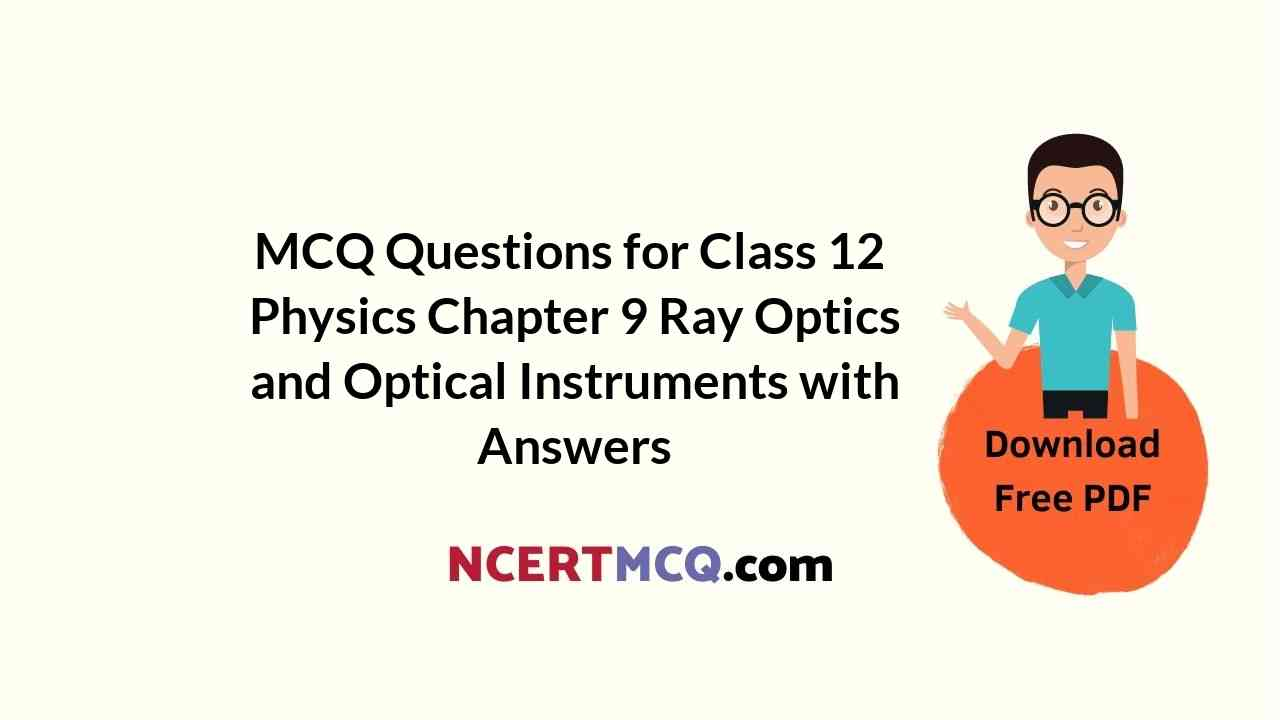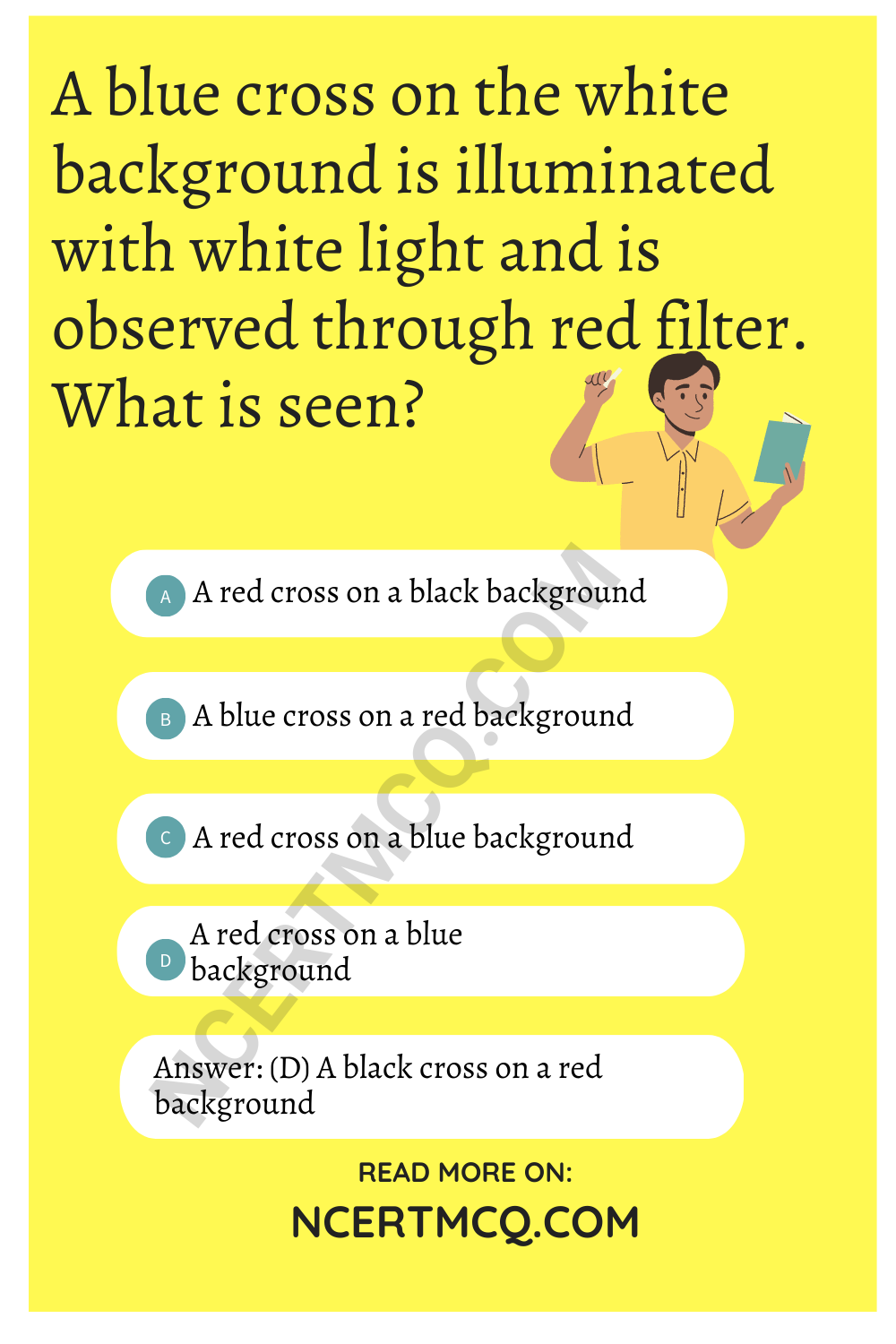Check the below NCERT MCQ Questions for Class 12 Physics Chapter 9 Ray Optics and Optical Instruments with Answers Pdf free download. MCQ Questions for Class 12 Physics with Answers were prepared based on the latest exam pattern. We have provided Ray Optics and Optical Instruments Class 12 Physics MCQs Questions with Answers to help students understand the concept very well.

## Ray Optics and Optical Instruments Class 12 MCQs Questions with Answers

Ray Optics Class 12 MCQ Question 1.
Which of-the following is not a property of light?
(a) It can travel through vacuum
(b) It has a finite speed
(c) It requires a material medium for its propagation
(d) It involve transportation energy

Answer: (c) It requires a material medium for its propagation

MCQ On Ray Optics Class 12 Question 2.
Two points P and q are situated at the same distance from a source of light but on opposite sides. The plane difference between the light waves passing through P and q will be:
(a) n
(b) 2n
(c) $$\frac{π}{2}$$
(d) zero

MCQ On Reflection Of Light Class 12 Question 3.
The phase difference between the electric and the magnetic field vectors in electromagnetic waves is
(a) $$\frac{π}{4}$$
(b) $$\frac{π}{2}$$
(c) π
(d) zero

MCQ Of Ray Optics Class 12 Question 4.
A ray of light travelling in air is incident of a glass slab. The ray gets partly reflected and partly refracted. The phase difference between the reflected and the refracted waves is :
(a) $$\frac{π}{2}$$
(b) $$\frac{π}{4}$$
(c) π
(d) zero

Ray Optics Class 12 MCQs With Answers Question 5.
Which of the following cannot be polarised?
(b) transverse waves
(c) sound waves
(d) X-rays

Ray Optics MCQ Questions Pdf Question 6.
Light year is the unit of:
(a) distance
(b) time
(c) intensity of light
(d) None of these

Ray Optics Objective Questions Question 7.
If a mirror is approaching you at a speed of 10 ms^1, the speed with which your image approach you is:
(a) 10 ms-1
(b) 5 ms-1
(c) 20 ms-1
(d) 15 ms-1

MCQ On Ray Optics Class 12 Pdf Question 8.
The refractive indices (R.I.) of glass and water with respect to air are 3/2 and 4/3 respectively. The R.I. of glass w.r. to w ater is:
(a) 8/9
(b) 9/8
(c) 7/6
(d) 2

Ray Optics MCQ With Answers Question 9.
The angle of minimum deviation for an equilateral glass prism is 30°. Refractive index of the prism is:
(a) $$\frac{1}{√3}$$
(b) √2
(c) 1
(d) can’t be determined

MCQ On Optics Class 12 Question 10.
A beam of light is incident at 60° to a plane surface. The reflected and refracted rays are perpendicular to each other. What is the R.I. of the surface:
(a) $$\frac{1}{√3}$$
(b) √3
(c) $$\frac{1}{3}$$
(d) 3

Optics MCQ With Answers Question 11.
A concave mirror of focal length f produces an image n times the size of the object. If the image is real then the distance of the object is:
(a) (n – 1) f
(b) (n + 1) f
(c) ($$\frac{n+1}{n}$$)f
(d) ($$\frac{n-1}{n}$$)f

Answer: (c) ($$\frac{n+1}{n}$$)f

Ray Optics Class 12 MCQs Question 12.
A convergent lens will become less convergent in :
(a) oil
(b) water
(c) both of (a) and (b)
(d) none of these

Optics Class 12 MCQ Question 13.
A thin glass (RI = $$\frac{3}{2}$$) lens has optical power of – 5D in air. Its optical power in a liquid medium with RI = 1.6 will be:
(a) -2.5 D
(b) 25 D
(c) -ID
(d) 0.625 D

Optics MCQs Class 12 Question 14.
On reflection from a denser medium, a light wave suffers a phase difference of:
(a) π
(b) $$\frac{π}{2}$$
(c) $$\frac{π}{4}$$
(d) 2π

MCQ Questions Of Ray Optics Class 12 Question 15.
The branch of optics dealing with the formation of images using the concept of straight line propagation of light is called:
(a) geometrical optics
(b) physical optics
(c) corpuscular optics
(d) quantum optics

Question 16.
When light travels from one medium to another, which of the following does not change?
(a) frequency
(b) refractive index
(c) velocity
(d) wavelengthQuestion 17.
Two lens of power -15 D and +5 D are placed in contact co-axially. The focal length of equivalent lens is:
(a) -10 cm
(b) -20 cm
(c) +10 cm
(d) None

Question 18.
Refractive index of water and glass are $$\frac{4}{3}$$ and $$\frac{5}{3}$$. A light ray is going to water from glass. Then, its critical angle will be:
(a) sin-1$$\frac{4}{5}$$
(b) sin-1$$\frac{5}{6}$$
(c) sin-1$$\frac{1}{2}$$
(d) sin-1($$\frac{2}{1}$$)

Answer: (b) sin-1$$\frac{5}{6}$$

Question 19.
The air bubble inside water shine due to:
(a) Reflection
(b) Refraction
(c) T.l.R.
(d) None of these

Question 20.
When a ray of light (white) enters a lens, undergoes a change is:
(a) wavelength
(b) velocity
(c) frequency
(d) both velocity and wavelength

Answer: (d) both velocity and wavelength

Question 21.
Radio waves from an antenna travel with the velocity of:
(a) light
(b) sound
(c) ultrasonics
(d) supersonics

Question 22.
A point object is placed at the centre of a glass sphere of radius 6 cm 3 and RI = $$\frac{3}{2}$$. The distance of virtual image from the surface of the sphere is :
(a) 2 cm
(b) 4 cm
(c) 6 cm
(d) 12 cm

Question 23.
The RI. of the material of a prism is √2 and its refracting angle is 30°. One of the refracting surface of the prism is made a mirror. A beam of monochromatic light entering the prism from the other face retraces its path, after reflection from mirror surface. The angle of incidence on prism is:
(a) 0°
(b) 30°
(c) 45°
(d) 60°

Question 24.
A telescope has an objective lens of 10 cm diameter and is situated at a distance of 1 km from two objects. The minimum distance between these two objects, which can be resolved by the telescope, when seen in the light of mean λ = 5000 A is of the order of:
(a) 5 mm
(b) 5 cm
(c) 0.5 m
(d) 5 m

Question 25.
When a beam of light is used to determine the position of an object, the maximum accuracy is achieved if the light is :
(a) polarized
(b) of longer λ
(c) of shorter λ
(d) of high intensity

Question 26.
A convex lens is dipped in a liquid, whose R.I. is equal to that of R.I. of material of the lens. Then its focal length will:
(a) become zero
(b) become infinite
(c) reduce
(d) increase

Question 27.
The velocity of light in vacuum is 3 × 1010 cms-1. The velocity of light in a medium (µ = 1.5) is:
(a) 2 × 1010cms-1
(b) 4.5 × 1010cms-1
(c) 1.5 × 1010cms-1
(d) None of these

Question 28.
Which of the following is not essential for the formation of a pure spectrum?
(a) parallel beam of light
(b) narrow beam of light
(c) screen
(d) prism in the minimum deviation position

Question 29.
A certain far-sighted person cannot see objects closer to the eye than 100 cm. The power of the lens which will enable him to read at a distance of 25 cm will be?
(a) 3D
(b) 1D
(c) 4D
(d) 2D

Question 30.
Which produces additional luminiscence in a fluorescent tube?
(a) infra red rays
(b) ultraviolet rays
(c) micro waves

Question 31.
For achromatic combination of lenses, if we use two lenses of focal length f’ and f1 dipersive power to ω and ω’ respectively, then
(a) ω = ω0, ω’= 2ω0, f’ = 2f
(b) ω = ω0, ω’ = 2ω0, f’ = -2f
(c) ω = ω0, ω’ = 2ω0, f’ = f/2
(d) ω = ω0, ω’ = 2ω0, f’ = -f/2

Answer: (d) ω = ω0, ω’ = 2ω0, f’ = -f/2

Question 32.
ar point of a myopic eye is 40 cm. The dioptic power of a corrective lens is:
(a) 40 D
(b) -4 D
(c) -2.5 D
(d) -0.25 diopter

Question 33.
Two thin lenses of focal lengths 20 cm and 25 cm are placed in contact. The effective power of the combination is:
(a) 45 D
(b) 9 D
(c) $$\frac{1}{9}$$ D
(d) 6 D

Question 34.
A blue cross on the white background is illuminated with white light and is observed through red filter. What is seen?
(a) A red cross on a black background
(b) A blue cross on a red background
(c) A red cross on a blue background
(d) A black cross on a red background

Answer: (d) A black cross on a red backgroundQuestion 35.
The angle of deviation for a prism is greatest for:
(a) violet
(b) red
(c) orange
(d) yellow

Question 36.
A double convex air bubble in water behaves as:
(a) Convergent lens
(b) divergent lens
(c) plane slab
(d) concave mirror